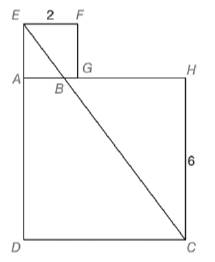Chapter 5.2, Problem 40EElementary Geometry For College St...

7th Edition
Alexander + 2 others
ISBN: 9781337614085

Solutions

Chapter
SectionElementary Geometry For College St...

7th Edition
Alexander + 2 others
ISBN: 9781337614085
Textbook Problem

For Exercises 39 to 40, use intuition to from a proportion based on the drawing shown.A square with sides of length 2 in. rests (as shown) on a square with sides of length 6 in. Find the perimeter of trapezoid A B C D .To determine

To calculate:

The perimeter of trapezoid ABCD

Explanation

Given:

A square with sides of length 2in. rests on a square with sides of length 6in.

Definition Used:

If C is a point of XY¯ and XCY, then XC+CY=XY.

Square:

It is a four sides of regular polygon where all sides are equal and all the internal angle equal to 90.

Similarity between Two polygons:

Two polygons are similar if and only if when all pairs of corresponding angles are congruent and all pairs of corresponding sides are proportional.

Pythagoras Theorem:

It states that sum of the length of the two sides is equal to the square of the length of the hypotenuse (longest sides).

(Hypotenuse)2=(Base)2+(Perpendicular)2

Perimeter of Polygon:

It is the total distance around the outside of a polygon.

Calculation:

According to the segment-Addition Postulate, AB+BH=AH.

Let us assume that AB=x.

It is given that AHCDandAGFE is a square.

According to the property of a square, we can say that AH=HC=CD=DA=6in and AG=GF=FE=EA=2in.

Substitute the value of AHandAB in an equation AB+BH=AH, we get

x+BH=6BH=6x

By the condition of two similar polygon, EAHC=ABBH.

So,

26=x6x13=x6x6x=3x4x=6x=64x=32

So, AB=32

Still sussing out bartleby?

Check out a sample textbook solution.

See a sample solution

The Solution to Your Study Problems

Bartleby provides explanations to thousands of textbook problems written by our experts, many with advanced degrees!

Get Started

By comparing areas, show that 12+13++1nlnn1+12+13++1n1

Single Variable Calculus: Early Transcendentals, Volume I

Expand each expression in Exercises 122. (2y+3)(y+5)

Finite Mathematics and Applied Calculus (MindTap Course List)

Find the limit. limx1sin(x1)x2+x2

Calculus: Early Transcendentals